JEE  >  Test: Matrices- 2

# Test: Matrices- 2

Test Description

## 25 Questions MCQ Test Mathematics (Maths) Class 12 | Test: Matrices- 2

Test: Matrices- 2 for JEE 2023 is part of Mathematics (Maths) Class 12 preparation. The Test: Matrices- 2 questions and answers have been prepared according to the JEE exam syllabus.The Test: Matrices- 2 MCQs are made for JEE 2023 Exam. Find important definitions, questions, notes, meanings, examples, exercises, MCQs and online tests for Test: Matrices- 2 below.
Solutions of Test: Matrices- 2 questions in English are available as part of our Mathematics (Maths) Class 12 for JEE & Test: Matrices- 2 solutions in Hindi for Mathematics (Maths) Class 12 course. Download more important topics, notes, lectures and mock test series for JEE Exam by signing up for free. Attempt Test: Matrices- 2 | 25 questions in 25 minutes | Mock test for JEE preparation | Free important questions MCQ to study Mathematics (Maths) Class 12 for JEE Exam | Download free PDF with solutions
 1 Crore+ students have signed up on EduRev. Have you?
Test: Matrices- 2 - Question 1

###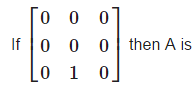Detailed Solution for Test: Matrices- 2 - Question 1

A square matrix A for which An= 0 , where n is a positive integer, is called a Nilpotent matrix.

Test: Matrices- 2 - Question 2

###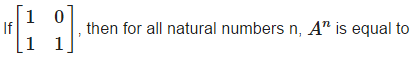Detailed Solution for Test: Matrices- 2 - Question 2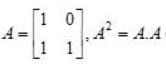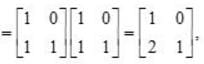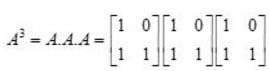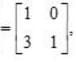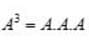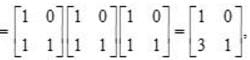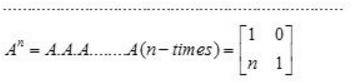Test: Matrices- 2 - Question 3

### If A any square matrix then which of the following is not symmetric ?

Detailed Solution for Test: Matrices- 2 - Question 3

For every square matrix (A – A’) is always skew – symmetric.

Test: Matrices- 2 - Question 4

Let a, b, c, d, u, v be integers. If the system of equations, a x + b y = u, c x + dy = v, has a unique solution in integers, then

Detailed Solution for Test: Matrices- 2 - Question 4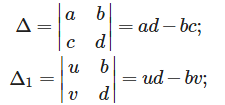ax + by = u , cx +dy = v ,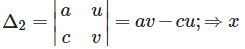since the solution is unique in integers.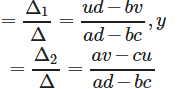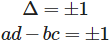Test: Matrices- 2 - Question 5

The system of equations, x + y + z = 1, 3 x + 6 y + z = 8, αx + 2 y + 3z = 1 has a unique solution for

Detailed Solution for Test: Matrices- 2 - Question 5

The given system of equations has unique solution , if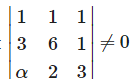⇒1(18−2)−1(9−α) ⇒13−5α ≠ 0 ⇒ α ≠ 13/5 + 1(6−6α) ≠ 0
Therefore , unique solution exists for all integral values of α.

Test: Matrices- 2 - Question 6

If A and B are symmetric matrices of the same order, then

Detailed Solution for Test: Matrices- 2 - Question 6

If A and B are symmetric matrices of the same order, then , AB + BA is always a symmetric matrix.

Test: Matrices- 2 - Question 7

If A = [aij]2×2 where aij= i + j, then A is equal to

Detailed Solution for Test: Matrices- 2 - Question 7

If A = [aij]2x2 where aij = i + j, then,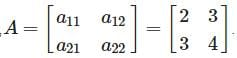Test: Matrices- 2 - Question 8

Each diagonal element of a skew-symmetric matrix is

Detailed Solution for Test: Matrices- 2 - Question 8

The diagonal elements of a skew-symmetric is zero.

Test: Matrices- 2 - Question 9

The system of equations, x + y + z = 6, x + 2 y + 3 z = 14, x + 3 y + 5z = 20 has

Detailed Solution for Test: Matrices- 2 - Question 9

The given system of equations does not has a solution if :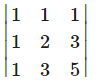0 ⇒ 1(10 -9) - 1(5-3) + 1(3-2)

= 0 ⇒ 1-2 + 1 = 0

Test: Matrices- 2 - Question 10

The matrix of the transformation ‘reflection in the line x + y = 0 ‘ is

Detailed Solution for Test: Matrices- 2 - Question 10

Let x' and y' be the reflection of x and y, therefore :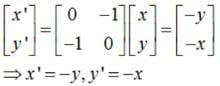Hence, reflection is on the line - x-y = 0⇒ x + y = 0

Test: Matrices- 2 - Question 11

A square matrix A = [aij]n×n is called a diagonal matrix if aij = 0 for

Detailed Solution for Test: Matrices- 2 - Question 11

In a diagonal matrix all elements except diagonal elements are zero.i.e.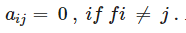Test: Matrices- 2 - Question 12

If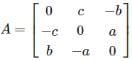and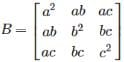then AB =

Detailed Solution for Test: Matrices- 2 - Question 12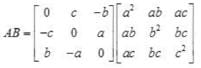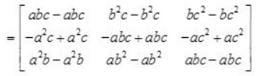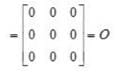Test: Matrices- 2 - Question 13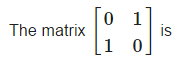Detailed Solution for Test: Matrices- 2 - Question 13

or a symmetric matrix A’ = A . therefore ,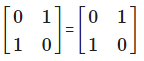Test: Matrices- 2 - Question 14

If In is the identity matrix of order n, the (In)−1

Detailed Solution for Test: Matrices- 2 - Question 14

Inverse of any identity matrix is always an identity matrix.

Test: Matrices- 2 - Question 15

If A = [x y z],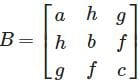and C = [xyt]t, then ABC is

Detailed Solution for Test: Matrices- 2 - Question 15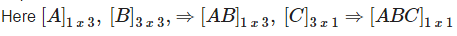Test: Matrices- 2 - Question 16

If a square matrix A has two identical rows or columns , then det.A is :

Detailed Solution for Test: Matrices- 2 - Question 16

Det.A = 0.

Test: Matrices- 2 - Question 17

For a skew symmetric odd ordered matrix A of integers, which of the following will hold true:

Detailed Solution for Test: Matrices- 2 - Question 17

Determinant of a skew symmetric odd ordered matrix A is always 0 .

Test: Matrices- 2 - Question 18

Matrix A when multiplied with Matrix C gives the Identity matrix I, what is C?

Detailed Solution for Test: Matrices- 2 - Question 18

Any square matrix when multiplied with its inverse gives the identity matrix. Note that non square matrices are not invertible.

Test: Matrices- 2 - Question 19

Let for any matrix M ,M−1exist. Which of the following is not true.

Detailed Solution for Test: Matrices- 2 - Question 19

Clearly , (M−1)−1 = (M−1)1 is not true.

Test: Matrices- 2 - Question 20

Rank of a non-zero matrix is always

Detailed Solution for Test: Matrices- 2 - Question 20

Rank of a non zero matrix is always greater than or equal to 1.

Test: Matrices- 2 - Question 21

For a non-trivial solution | A | is

Detailed Solution for Test: Matrices- 2 - Question 21

An n×n homogeneous system of linear equations has a unique solution (the trivial solution) if and only if its determinant is non-zero. If this determinant is zero, then the system has an infinite number of solutions. i.e. For a non-trivial solution ∣A∣=0.

Test: Matrices- 2 - Question 22

If for a matrix A, A2+I = O where I is the identity matrix, then A equals

Detailed Solution for Test: Matrices- 2 - Question 22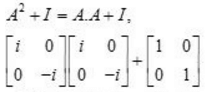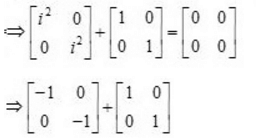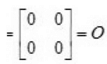Test: Matrices- 2 - Question 23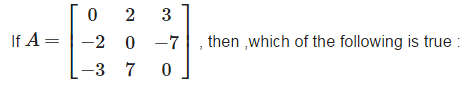Detailed Solution for Test: Matrices- 2 - Question 23

The given matrix is a skew – symmetric matrix.,therefore , A = - A’.

Test: Matrices- 2 - Question 24

The system of linear equations x + y + z = 2, 2x + y - z = 3, 3x + 2y - kz = 4 has a unique solution if ,

Detailed Solution for Test: Matrices- 2 - Question 24

The given system of equation has a unique solution if :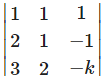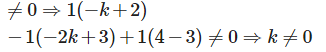Test: Matrices- 2 - Question 25

The value of k for which the system of equations, x + k y + 3 z = 0, 3 x + k y – 2 z = 0, 2 x + 3 y – 4 z = 0, have a non-trival solution is

Detailed Solution for Test: Matrices- 2 - Question 25

The given system of equations has a non-trivial solution if  :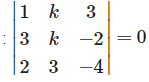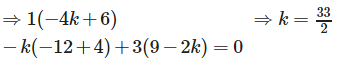## Mathematics (Maths) Class 12

209 videos|218 docs|139 tests
Information about Test: Matrices- 2 Page
In this test you can find the Exam questions for Test: Matrices- 2 solved & explained in the simplest way possible. Besides giving Questions and answers for Test: Matrices- 2, EduRev gives you an ample number of Online tests for practice

## Mathematics (Maths) Class 12

209 videos|218 docs|139 tests(Scan QR code)